﻿ Dynamic Directional NxN Chart Based Text Based Substitution CipherPublications are Open
Access in this journal
Article Versions
Export Article
• Normal Style
• MLA Style
• APA Style
• Chicago Style
Research Article
Open Access Peer-reviewed

### Dynamic Directional NxN Chart Based Text Based Substitution Cipher

Michael M Kangethe , Elisha Odira Abade
Journal of Computer Sciences and Applications. 2020, 8(2), 56-61. DOI: 10.12691/jcsa-8-2-3
Received August 11, 2020; Revised September 14, 2020; Accepted September 23, 2020

### Abstract

The need for simple secure substitution encryption algorithms has risen due to complexities derived from current encryption algorithms, which over-complicate the process through many sub-processes and consume more space than necessary. This also creates the need for a secure string encryption approach that can also be applied using a simple pen and paper to encrypt and decrypt messages between each other. In this paper we present a technique based on a Non-Deterministic directional NxN chart-based encryption cipher as a solution to this problem. This will be achieved by using a 2-dimensional N by N chart as the key while using a dynamically selected directional approach to generate both the cipher and plain-text.

### 1. Introduction

Cryptography is the practice and method for secure communication in the presence of third parties called adversaries. (Tanmoy and Ramkrishna, 2015)

Implementation of simple secure ciphers for text-based communication has become a necessity for efficiency of communication unlike other digital forms of communication. This has led to the development of several text-based ciphers based for this purpose.

The development of a randomized substitution cipher enhances the security of the cipher by obscuring the process of encryption and decryption of the data making it nearly impossible to decipher the data. This paper demonstrates how randomization can be used as a principle in substitution ciphers to enhance their security through the use of directional X, Y chart key-based character substitution.

### 2. Background Concept

Substitution is the replacement of one character with another. By using current text substitution ciphers the security of the data is usually weaker due to the limited number of possible representations of the ciphertext based on the plaintext. This forces cryptographers to build encryption algorithms that use multiple ciphers together with the substitution cipher primitive to strengthen the security of their developed encryption algorithms.

Randomization is a selection method base on pure chance alone and has been a reliable security concept when applied to cryptography as it introduces greater complexities to the process of encryption and decryption. The current known popular approaches of randomness apply in the generation of IVs. And recently in the use of encryption primitives.

Randomized text encryption algorithm proposed increases the complexity of cryptanalyst to decrypt the ciphertext and restricts them to break the security of encoded file. The proposed technique uses random numbers added to plaintext along with encryption key. After applying encryption technique, each time same plaintext will be converted to different ciphertext provided that encryption key is same or different 3.

Message Based Random Variable Length Key Encryption Algorithm. Dynamic and message dependent key generator was created by producing a random number and it was selected as the size of first chunk. Residual value of second chunk divided by first chunk concatenating with first chunk forms the first cipher as an input for SP-boxes. These processes repeated until whole message get involved into the last cipher. Encrypted messages are not equal under different run. Value of random number should be greater than 35 bits and plaintext must be at least 7 bits. A padding algorithm was used for small size messages or big random numbers 4.

Probabilistic Encryption aims to provide a practical implementation of a probabilistic cipher by extending on the algorithms by Fuchsbauer, Goldwasser and Micali. We provide details on designing and implementing the cipher and further support our understanding by providing a statistical analysis of our implementation for the key generation, encryption, and decryption times taken by the cipher for key sizes of 1024, 2048, and 4096 bits for varying message spaces of 750, 1500, 3000, and 5000 bits. The concept of 'inter-bit operating time' is introduced for the cipher which calculates time elapsed between two instances of an operation 6.

### 3. Proposed Algorithm

The main approach to the solution is to create a substitution Cipher that uses a dynamically Generated Key Chart with randomly generated substitution values. Then use the Key Chart to navigate randomly in an X, Y direction to select the cipher substitution value for each plaintext character. The process should be reversible thus one should be able to Encrypt and decrypt the data given the Key Chart and the Directional sequence as the key.

In the encryption process only, the plaintext will be needed. The output of the encryption process will be three things namely:

1. Key Chart

2. Direction Sequence

3. Ciphertext

Both the Key Chart and the Direction Sequence can be combined to form a single key which will be used to reverse the encryption process and decrypt the ciphertext to attain the plaintext.

We assume the plaintext to be encrypted is in alphanumeric (A-Z,0-9) the first step will be to create a key chart that will contain the corresponding values of each character. This key chart will be used for the encryption and decryption process.

This is represented as mathematical process below:

3.1.1. Encryption Process

Given a 2 Dimension Chart C made of N by N hexadecimal character space we first get.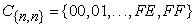Equation 1: Substitution Chart table Character space

And for each plaintext character pi in the input text P the output Ei will become: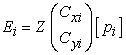Equation 2: Substitution formular for each character in the Plaintext

Where Z is the Random Selection with respect to the substitution Chart’s X and Y coordinates.

Using the chart as the substitution reference we follow the below rule to define the encryption process: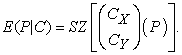Equation 3: The General Substitution formular for the Plaintext

Where:

E(P | C) is the Encryption of the plaintext P with respect to the substitution Chart C.

S is the substitution process

The output will be the ciphertext E and Substitution process stored as KS.

3.1.2. Decryption Process

Using the generated substitution mapping key from the process and stored as a key Ks we reverse the process by using the following process: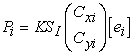Equation 4: Substitution formular for each character in the Ciphertext

Where ei is the encrypted character at index i of the encrypted text E.

Thus, giving us the general formular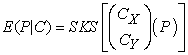Equation 5: The General Reverse Substitution formular for the Ciphertext

For the purpose of demonstration, the Algorithm will be described using a real-world example as detailed below

3.2. Example

Using the table below one should be able to encrypt my message 2341 For demonstration purpose we will use a small table of only four characters as our Key-space.

The first row and column represent the plain-text mappings.

The data inside the table are the corresponding substituted representations of the plain-text data items at each instance.

3.2.1. Key-Chart Requirements

The column items have been placed in reverse unlike order to the rows so as to eliminate the possibility of cipher collision which is a case where multiple cipher approaches will lead to the same plain-text. This has also been applied at cipher-text key items Ki in the chart although the order is the same except at each row the item Ki appears at the Ki-1 with full rotation for the outlying characters.

3.2.1.1. Note

Ÿ The first row will contain a random placement of all characters from in the alphanumeric set.

Ÿ The subsequent rows will contain the same set but with the characters shifted one position to the left or right.

Ÿ The overflowing keys will be placed in the starting points and this should follow until the key space cycle is complete.

3.2.2. Encryption

Assuming the system selected the second row at random and the directions selected was H, V, H, V where H represents the Horizontal direction and V represents the vertical directions. This can also be represented as V=1 and H=0.

Therefore Using 2341 as our plain-text we encrypt it to become.

Steps:

Ÿ 2 will correspond to C [At column 2 and row 3]

Ÿ 3 will correspond to C [At column 2 and row 3] since we are selecting the corresponding row id Vertically,

Ÿ 4 will correspond to A [At column 4 and row 3]

Ÿ And finally, 1 will correspond to C [At column 4 and row 1]

So, the cipher-text 2341 will become CCAC.

3.2.3. Decryption

From the cipher-text above there is enough diffusion to eliminate its association to the plain-text

To decrypt the same text, we will need the chart and starting point which is H2 and the decryption process is represented as H, V, H, V

Therefore, Using CCAC as our cipher-text we Decrypt it to become.

Steps:

Ÿ C will correspond to the Column Key 2[At column 2 and row 3]

Ÿ C will correspond to the Row Key 3[At column 2 and row 3]

Ÿ A will correspond to the Column Key 4[At column 4 and row 3]

Ÿ and finally, C will correspond to the Row 1 Key 2[At column 4 and row 1]

Finally, the plain-text using the same hey and direction process of CCAC will become 2341.

3.3. The Pseudo Algorithm

Variables

Random_Choices = “X”,”Y”

Direction_Selections[] =null

Cipher-text[] =null

3.3.1. Encryption Function

Function Encrypt():

{

Get Key_Chart,Current_x, Current_y,input_String

For i in input_String

Direction_Selections[i]=GenerateRandomChoice(“X”,”Y”)

if(Direction_Selections[i] == “X”)

Cipher-text[i] = Encrypt_Horizontal(Key_Chart,Current_x,Current_y,input_String[i])

Else

Cipher-text[i] = Encrypt_Vertical(Key_Chart,Current_x,Current_y,input_String[i])

}

3.3.2. Decryption Function

Function Decrypt():

{

Get Key_Chart,Current_x, Current_y,input_String,Direction_Selections[]

For i in input_String

if(Direction_Selections[i] == “X”)

Cipher-text[i] = Decrypt_Horizontal(Key_Chart,Current_x,Current_y,input_String[i])

Else

Cipher-text[i] = Decrypt_Vertical(Key_Chart,Current_x,Current_y,input_String[i])

}

3.3.3. X AND Y Encryption Functions

function Encrypt_Horizontal(Key_Chart,Current_x,Current_y,Prev_Y,Key)

{

For i in Key_Chart:

if(Key_Chart[i].equals(Key))

{

Current_x =i;

Current_y=Prev_Y;

Temp = Key_Chart[Current_y][i];

break;

}

Return Temp

}

function Encrypt_Vertical(Key_Chart,Current_x,Current_y,Prev_Y,Key)

{

For i in Key_Chart:

if(Key_Chart[i].equals(Key))

{

Current_x =X;

Current_y=i;

Temp = Key_Chart[i][Current_x];

break;

}

Return Temp

}

3.3.4. X AND Y Encryption Functions

function : Decrypt_Vertical(Key_Chart,Current_x,Current_y,Prev_Y,Key)

{

For i in Key_Chart:

if(Key_Chart[i][Current_x].equals(Key))

{

X_pos =Current_x;

Y_pos=i;

Temp =Key_Chart[i];

}

Return Temp

}

function Decrypt_Horizontal(Key_Chart,,Current_y,Prev_Y,Key)

{

For i in Key_Chart:

if(Key_Chart[Current_y][i].equals(Key))

{

X_pos =i;

Y_pos=Current_y;

Temp =Current_y[i];

}

Return Temp

}

3.4. Security

To determine the algorithms security against brute force it was necessary to consider in the below factors.

3.4.1. Size of Data N

Key-space this is the Chart of Characters in the cipher in the PoC ranging from 0-9 and A-Z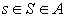Complexity is calculated using the below factors:

For a sentence S comprising of characters s0, s1, s2, ......, sn-1, sn.

For every si in S complexity is n where n is the size of data N at each subset.

Thus, the complexity for the string is

Complexity =S2.

3.5. Proof of Security
3.5.1. Crypt-analysis through brute-force of Possible Matches

Given 4 data items as the plain-text and cipher and 4 Possible Directions and 8 possible starting points we can use 0 to represent H and 1 to represent V and a binary table to list the possible outcomes as below.

Assuming we start with the first C in the first row as our default

From the above proof of tables, it is evident that for each combination of cipher-text there are N2 possible plain-text options which increases the complexity of deciphering the text by itself. Where N is the size of the Cipher/Plain-text.

The correct plain-text from the cipher-text in the table also appears only once as highlighted in the second table. Thus, ensuring there won’t be any collisions when deciphering the data.

### 4. Assumptions and Limitations of Scope

For the purpose of demonstration, the research and development of this algorithm focused solely on the decoded alphanumeric substitution process and not in any other extended digital data format and encoding or encryption primitives.

### 5. Conclusion

In this paper, we proposed an algorithm to encrypt the data based on random and dynamic Key Chat generation and substitution. The above system is produces reliable and stable results. However, the system can be improved by using Hex values for all types of data encryption. Keeping this in mind, the algorithm has been designed in a quite simple manner while focusing on high security.

### References

  M. A. Murillo-Escobar, F. Abundiz-PÈrez, C. Cruz-Hern·ndez, R. M. LÛpez-GutiÈrrez. 2014. A novel symmetric text encryption algorithm based on logistic map. In article  Mante, Pratik Gajanan, Oswal, Harsh Rajendra, Swain, Debabrata, Deshpande, Deepali. 2009. A Symmetrical Encryption Technique for Text Encryption Using Randomized Matrix Based Key Generation. In article  Jamshed Memon, Mohd Zaidi Abd Rozan, Mueen Uddin, Adamu Abubakar, Haruna Chiroma, Dzurllkanian Daud. 2014. Randomized Text Encryption: a New Dimension in Cryptography. International Review on Computers and Software (I.RE.CO.S.), Vol. 9, N. 2 In article View Article  Hamid Mirvaziri, Kasmiran Jumari Mahamod Ismail and Zurina Mohd Hanapi. 2009. Message Based Random Variable Length Key Encryption Algorithm. Journal of Computer Science 5 (8): 573-578, 2009 In article View Article  Dhananjay S. Phatak, Qiang Tang, Alan T. Sherman, Warren D. Smith, Peter Ryan, † Kostas Kalpakis. 2014. DoubleMod and SingleMod: Simple Randomized Secret-Key Encryption with Bounded Homomorphicity In article  Orhio Mark Creado, Yiling Wang, Xianping Wu, and Phu Dung Le. 2009. Probabilistic Encryption – A Practical Implementation, Fourth International Conference on Computer Sciences and Convergence Information Technology. In article View ArticleThis work is licensed under a Creative Commons Attribution 4.0 International License. To view a copy of this license, visit http://creativecommons.org/licenses/by/4.0/